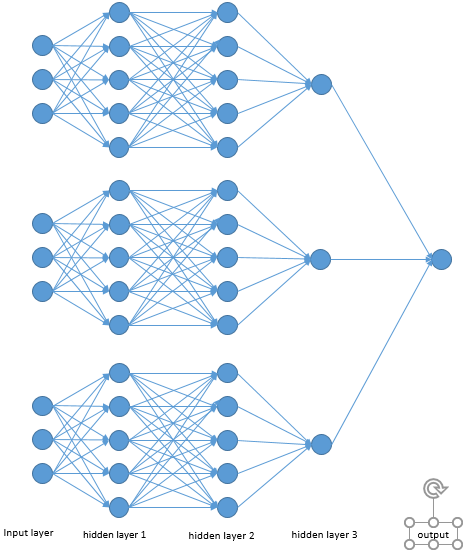# Reconstruct images from PCA reduced dimensions with NN

I was reading this Medium post and I had the idea to reconstruct the original images with a convolutional neural network instead of applying the inverse transform method. The problem is that I don't know how to define the input of the CNN. From what I know, PCA reconstructs the original images as a linear combination of the eigenvectors, so I guess the features should be the k eigenvectors for each original image, but having the same features for different labels doesn't make sense. Is it possible to recontruct the images from the reduced dimensions? If possible, what approach is the one you think would work?

I'm not sure I understand your question. You want to create a neural network able to reconstruct an image thanks to its PCA ? This could be some sort of autoencoder that uses PCA for its latent representation. The encoder of the AE would simply be the PCA (AE isn't actually relevant here, nothing trainable in the "encoder", but I like the parallel since we're talking about generative machine learning/up-scaling 2D representations).

If possible, what approach is the one you think would work?

Since an image will have 2D eigenvectors of already good shape, you could simply concatenate them, flatten, use a mean, use a concatenation of different 2D statistics/transformations, combine the results of several sub-networks starting from different 2D statistics of the eigenvectors (or the eigenvectors themselves), etc. as an intermediate representation. This is the tricky choice, following which you'll have to deal with typical AE decoder architecture design.

An illustration of the sub-networks idea (replace 1D inputs and 1D output with 2D data in your case):Is it possible to recontruct the images from the reduced dimensions?

If you're starting from reduced dimensions, it's not a problem: you can use either the so-called "deconvolution" (transposed convolution is the actual name) for direct 2D upscaling (see here for Keras implementation, and here for the equivalent PyTorch function), or simply if working with a flattened input/intermediate representation, re-shape a 1D large enough neural network layer, to go back to the original image shape.

Finally, note that if you use a neural network as it is commonly defined, your transformations will involve non-linearities.

That being said, not sure it's going to give you a great result, worth a try ! IMO you should try feeding each (or the n-largest) eigenvector into their own very simple sub-network, before combining their respective intermediate representations in a common final network that will output the right shape. Use simple architectures since you probably want to compare with linear combinations of eigenvectors.